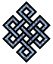fail2ban bad ip database: ip 110.185.106.47

| ip database | live view | stats | report | help | api key:

 ip: 110.185.106.47 hostname: 110.185.106.47 country:[CN] China first reported: 21.11.2018 02:34.23 GMT+0200 last reported: 15.03.2019 02:18.23 GMT+0200 time period: 113d 23h 44m total reports: 12 reported by: 1 host(s) filter(s): ssh (6) tor exit node no badips.com db Lookupport scan of '110.185.106.47':

[-hide]
```# Nmap 6.40 scan initiated Wed Nov 21 02:35:02 2018 as: /usr/bin/nmap -sU -sS -O 110.185.106.47
Nmap scan report for 110.185.106.47
Host is up (0.24s latency).
Not shown: 999 open|filtered ports, 993 filtered ports
PORT     STATE  SERVICE
22/tcp   open   ssh
1521/tcp closed oracle
3306/tcp closed mysql
3389/tcp closed ms-wbt-server
8899/tcp closed ospf-lite
9999/tcp open   abyss
500/udp  open   isakmp
No exact OS matches for host (If you know what OS is running on it, see http://nmap.org/submit/ ).
TCP/IP fingerprint:
OS:SCAN(V=6.40%E=4%D=11/21%OT=22%CT=1521%CU=%PV=N%G=Y%TM=5BF4B6AB%P=x86_64-
OS:pc-linux-gnu)SEQ(SP=104%GCD=1%ISR=10D%TI=Z%CI=I%TS=8)SEQ(SP=104%GCD=1%IS
OS:R=10D%TI=Z%TS=8)OPS(O1=M5B4ST11NW7%O2=M5B4ST11NW7%O3=M5B4NNT11NW7%O4=M5B
OS:4ST11NW7%O5=M5B4ST11NW7%O6=M5B4ST11)WIN(W1=7120%W2=7120%W3=7120%W4=7120%
OS:W5=7120%W6=7120)ECN(R=Y%DF=Y%TG=40%W=7210%O=M5B4NNSNW7%CC=Y%Q=)T1(R=Y%DF
OS:=Y%TG=40%S=O%A=S+%F=AS%RD=0%Q=)T2(R=N)T3(R=N)T4(R=Y%DF=Y%TG=40%W=0%S=A%A
OS:=Z%F=R%O=%RD=0%Q=)T5(R=Y%DF=Y%TG=40%W=0%S=Z%A=S+%F=AR%O=%RD=0%Q=)T6(R=Y%
OS:DF=Y%TG=40%W=0%S=A%A=Z%F=R%O=%RD=0%Q=)T7(R=Y%DF=Y%TG=40%W=0%S=Z%A=S+%F=A
OS:R%O=%RD=0%Q=)U1(R=N)IE(R=Y%DFI=N%TG=40%CD=S)

OS detection performed. Please report any incorrect results at http://nmap.org/submit/ .
# Nmap done at Wed Nov 21 02:36:43 2018 -- 1 IP address (1 host up) scanned in 101.28 seconds
```
```Σ = 83 | Δt = 0.0054750442504883s
```